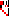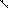# MINLPLib

### A Library of Mixed-Integer and Continuous Nonlinear Programming Instances

#### Instance: ex14_1_6

 Formatsⓘ ams gms lp mod nl osil pip Primal Bounds (infeas ≤ 1e-08)ⓘ 1.00000000 p1 ( gdx sol ) (infeas: 6e-17)0.00000000 p2 ( gdx sol ) (infeas: 4e-16) Other points (infeas > 1e-08)ⓘ Dual Boundsⓘ -0.00000000 (ANTIGONE)0.00000000 (BARON)0.00000000 (COUENNE)0.00000000 (LINDO)-0.00000003 (SCIP) Referencesⓘ Floudas, C A, Pardalos, Panos M, Adjiman, C S, Esposito, W R, Gumus, Zeynep H, Harding, S T, Klepeis, John L, Meyer, Clifford A, and Schweiger, C A, Handbook of Test Problems in Local and Global Optimization, Kluwer Academic Publishers, 1999.Kearfott, R and Novoa, M, Algorithm 681: INTBIS, A Portable Interval Newton/Bisection Package, ACM Transactions on Mathematical Software, 16:2, 1990, 152-157. Sourceⓘ Test Problem ex14.1.6 of Chapter 14 of Floudas e.a. handbook Added to libraryⓘ 31 Jul 2001 Problem typeⓘ QCP #Variablesⓘ 9 #Binary Variablesⓘ 0 #Integer Variablesⓘ 0 #Nonlinear Variablesⓘ 8 #Nonlinear Binary Variablesⓘ 0 #Nonlinear Integer Variablesⓘ 0 Objective Senseⓘ min Objective typeⓘ linear Objective curvatureⓘ linear #Nonzeros in Objectiveⓘ 1 #Nonlinear Nonzeros in Objectiveⓘ 0 #Constraintsⓘ 15 #Linear Constraintsⓘ 1 #Quadratic Constraintsⓘ 14 #Polynomial Constraintsⓘ 0 #Signomial Constraintsⓘ 0 #General Nonlinear Constraintsⓘ 0 Operands in Gen. Nonlin. Functionsⓘ Constraints curvatureⓘ indefinite #Nonzeros in Jacobianⓘ 60 #Nonlinear Nonzeros in Jacobianⓘ 32 #Nonzeros in (Upper-Left) Hessian of Lagrangianⓘ 14 #Nonzeros in Diagonal of Hessian of Lagrangianⓘ 8 #Blocks in Hessian of Lagrangianⓘ 5 Minimal blocksize in Hessian of Lagrangianⓘ 1 Maximal blocksize in Hessian of Lagrangianⓘ 3 Average blocksize in Hessian of Lagrangianⓘ 1.6 #Semicontinuitiesⓘ 0 #Nonlinear Semicontinuitiesⓘ 0 #SOS type 1ⓘ 0 #SOS type 2ⓘ 0 Infeasibility of initial pointⓘ 1 Sparsity JacobianⓘSparsity Hessian of Lagrangianⓘ```\$offlisting
*
*  Equation counts
*      Total        E        G        L        N        X        C        B
*         16        2        0       14        0        0        0        0
*
*  Variable counts
*                   x        b        i      s1s      s2s       sc       si
*      Total     cont   binary  integer     sos1     sos2    scont     sint
*         10       10        0        0        0        0        0        0
*  FX      0
*
*  Nonzero counts
*      Total    const       NL      DLL
*         62       30       32        0
*
*  Solve m using NLP minimizing objvar;

Variables  x1,x2,x3,x4,x5,x6,x7,x8,x9,objvar;

Equations  e1,e2,e3,e4,e5,e6,e7,e8,e9,e10,e11,e12,e13,e14,e15,e16;

e1..  - x9 + objvar =E= 0;

e2.. 0.004731*x1*x3 - 0.1238*x1 - 0.3578*x2*x3 - 0.001637*x2 - 0.9338*x4 + x7
- x9 =L= 0.3571;

e3.. 0.1238*x1 - 0.004731*x1*x3 + 0.3578*x2*x3 + 0.001637*x2 + 0.9338*x4 - x7
- x9 =L= -0.3571;

e4.. 0.2238*x1*x3 + 0.2638*x1 + 0.7623*x2*x3 - 0.07745*x2 - 0.6734*x4 - x7 - x9
=L= 0.6022;

e5.. (-0.2238*x1*x3) - 0.2638*x1 - 0.7623*x2*x3 + 0.07745*x2 + 0.6734*x4 + x7
- x9 =L= -0.6022;

e6.. x6*x8 + 0.3578*x1 + 0.004731*x2 - x9 =L= 0;

e7.. -x6*x8 - 0.3578*x1 - 0.004731*x2 - x9 =L= 0;

e8..  - 0.7623*x1 + 0.2238*x2 =E= -0.3461;

e9.. sqr(x1) + sqr(x2) - x9 =L= 1;

e10.. (-sqr(x1)) - sqr(x2) - x9 =L= -1;

e11.. sqr(x3) + sqr(x4) - x9 =L= 1;

e12.. (-sqr(x3)) - sqr(x4) - x9 =L= -1;

e13.. sqr(x5) + sqr(x6) - x9 =L= 1;

e14.. (-sqr(x5)) - sqr(x6) - x9 =L= -1;

e15.. sqr(x7) + sqr(x8) - x9 =L= 1;

e16.. (-sqr(x7)) - sqr(x8) - x9 =L= -1;

* set non-default bounds
x1.lo = -1; x1.up = 1;
x2.lo = -1; x2.up = 1;
x3.lo = -1; x3.up = 1;
x4.lo = -1; x4.up = 1;
x5.lo = -1; x5.up = 1;
x6.lo = -1; x6.up = 1;
x7.lo = -1; x7.up = 1;
x8.lo = -1; x8.up = 1;

Model m / all /;

m.limrow=0; m.limcol=0;
m.tolproj=0.0;

\$if NOT '%gams.u1%' == '' \$include '%gams.u1%'

\$if not set NLP \$set NLP NLP
Solve m using %NLP% minimizing objvar;

```

Last updated: 2021-07-09 Git hash: 827f1a2d